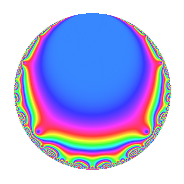# Properties

 Label 6034.2.a.hLevel 6034 Weight 2 Character orbit 6034.a Self dual Yes Analytic conductor 48.182 Analytic rank 1 Dimension 2 CM No Inner twists 1

# Related objects

## Newspace parameters

 Level: $$N$$ = $$6034 = 2 \cdot 7 \cdot 431$$ Weight: $$k$$ = $$2$$ Character orbit: $$[\chi]$$ = 6034.a (trivial)

## Newform invariants

 Self dual: Yes Analytic conductor: $$48.1817325796$$ Analytic rank: $$1$$ Dimension: $$2$$ Coefficient field: $$\Q(\sqrt{5})$$ Coefficient ring: $$\Z[a_1, \ldots, a_{5}]$$ Coefficient ring index: $$2$$ Fricke sign: $$1$$ Sato-Tate group: $\mathrm{SU}(2)$

## $q$-expansion

Coefficients of the $$q$$-expansion are expressed in terms of $$\beta = \sqrt{5}$$. We also show the integral $$q$$-expansion of the trace form.

 $$f(q)$$ $$=$$ $$q + q^{2} - q^{3} + q^{4} -\beta q^{5} - q^{6} - q^{7} + q^{8} -2 q^{9} +O(q^{10})$$ $$q + q^{2} - q^{3} + q^{4} -\beta q^{5} - q^{6} - q^{7} + q^{8} -2 q^{9} -\beta q^{10} + ( 2 + \beta ) q^{11} - q^{12} + ( 1 - \beta ) q^{13} - q^{14} + \beta q^{15} + q^{16} + ( -3 + \beta ) q^{17} -2 q^{18} + 3 q^{19} -\beta q^{20} + q^{21} + ( 2 + \beta ) q^{22} + ( -4 - \beta ) q^{23} - q^{24} + ( 1 - \beta ) q^{26} + 5 q^{27} - q^{28} + ( -1 + 2 \beta ) q^{29} + \beta q^{30} + ( -3 + 3 \beta ) q^{31} + q^{32} + ( -2 - \beta ) q^{33} + ( -3 + \beta ) q^{34} + \beta q^{35} -2 q^{36} + ( 2 + 4 \beta ) q^{37} + 3 q^{38} + ( -1 + \beta ) q^{39} -\beta q^{40} -2 \beta q^{41} + q^{42} + ( -2 + 4 \beta ) q^{43} + ( 2 + \beta ) q^{44} + 2 \beta q^{45} + ( -4 - \beta ) q^{46} + ( 2 - 2 \beta ) q^{47} - q^{48} + q^{49} + ( 3 - \beta ) q^{51} + ( 1 - \beta ) q^{52} + 3 q^{53} + 5 q^{54} + ( -5 - 2 \beta ) q^{55} - q^{56} -3 q^{57} + ( -1 + 2 \beta ) q^{58} -3 q^{59} + \beta q^{60} + ( -8 - 2 \beta ) q^{61} + ( -3 + 3 \beta ) q^{62} + 2 q^{63} + q^{64} + ( 5 - \beta ) q^{65} + ( -2 - \beta ) q^{66} + ( 3 - 3 \beta ) q^{67} + ( -3 + \beta ) q^{68} + ( 4 + \beta ) q^{69} + \beta q^{70} + ( -3 - 3 \beta ) q^{71} -2 q^{72} + ( -5 + \beta ) q^{73} + ( 2 + 4 \beta ) q^{74} + 3 q^{76} + ( -2 - \beta ) q^{77} + ( -1 + \beta ) q^{78} -12 q^{79} -\beta q^{80} + q^{81} -2 \beta q^{82} + ( -11 - \beta ) q^{83} + q^{84} + ( -5 + 3 \beta ) q^{85} + ( -2 + 4 \beta ) q^{86} + ( 1 - 2 \beta ) q^{87} + ( 2 + \beta ) q^{88} + ( -2 - 2 \beta ) q^{89} + 2 \beta q^{90} + ( -1 + \beta ) q^{91} + ( -4 - \beta ) q^{92} + ( 3 - 3 \beta ) q^{93} + ( 2 - 2 \beta ) q^{94} -3 \beta q^{95} - q^{96} + ( -2 + 3 \beta ) q^{97} + q^{98} + ( -4 - 2 \beta ) q^{99} +O(q^{100})$$ $$\operatorname{Tr}(f)(q)$$ $$=$$ $$2q + 2q^{2} - 2q^{3} + 2q^{4} - 2q^{6} - 2q^{7} + 2q^{8} - 4q^{9} + O(q^{10})$$ $$2q + 2q^{2} - 2q^{3} + 2q^{4} - 2q^{6} - 2q^{7} + 2q^{8} - 4q^{9} + 4q^{11} - 2q^{12} + 2q^{13} - 2q^{14} + 2q^{16} - 6q^{17} - 4q^{18} + 6q^{19} + 2q^{21} + 4q^{22} - 8q^{23} - 2q^{24} + 2q^{26} + 10q^{27} - 2q^{28} - 2q^{29} - 6q^{31} + 2q^{32} - 4q^{33} - 6q^{34} - 4q^{36} + 4q^{37} + 6q^{38} - 2q^{39} + 2q^{42} - 4q^{43} + 4q^{44} - 8q^{46} + 4q^{47} - 2q^{48} + 2q^{49} + 6q^{51} + 2q^{52} + 6q^{53} + 10q^{54} - 10q^{55} - 2q^{56} - 6q^{57} - 2q^{58} - 6q^{59} - 16q^{61} - 6q^{62} + 4q^{63} + 2q^{64} + 10q^{65} - 4q^{66} + 6q^{67} - 6q^{68} + 8q^{69} - 6q^{71} - 4q^{72} - 10q^{73} + 4q^{74} + 6q^{76} - 4q^{77} - 2q^{78} - 24q^{79} + 2q^{81} - 22q^{83} + 2q^{84} - 10q^{85} - 4q^{86} + 2q^{87} + 4q^{88} - 4q^{89} - 2q^{91} - 8q^{92} + 6q^{93} + 4q^{94} - 2q^{96} - 4q^{97} + 2q^{98} - 8q^{99} + O(q^{100})$$

## Embeddings

For each embedding $$\iota_m$$ of the coefficient field, the values $$\iota_m(a_n)$$ are shown below.

For more information on an embedded modular form you can click on its label.

Label $$\iota_m(\nu)$$ $$a_{2}$$ $$a_{3}$$ $$a_{4}$$ $$a_{5}$$ $$a_{6}$$ $$a_{7}$$ $$a_{8}$$ $$a_{9}$$ $$a_{10}$$
1.1
 1.61803 −0.618034
1.00000 −1.00000 1.00000 −2.23607 −1.00000 −1.00000 1.00000 −2.00000 −2.23607
1.2 1.00000 −1.00000 1.00000 2.23607 −1.00000 −1.00000 1.00000 −2.00000 2.23607
 $$n$$: e.g. 2-40 or 990-1000 Significant digits: Format: Complex embeddings Normalized embeddings Satake parameters Satake angles

## Inner twists

This newform does not admit any (nontrivial) inner twists.

## Atkin-Lehner signs

$$p$$ Sign
$$2$$ $$-1$$
$$7$$ $$1$$
$$431$$ $$-1$$

## Hecke kernels

This newform can be constructed as the intersection of the kernels of the following linear operators acting on $$S_{2}^{\mathrm{new}}(\Gamma_0(6034))$$:

 $$T_{3} + 1$$ $$T_{5}^{2} - 5$$ $$T_{11}^{2} - 4 T_{11} - 1$$